# Block and Spring in SHM with damping A block of mass 8.50 g on the end...

Block and Spring in SHM with damping

A block of mass 8.50 g on the end of spring undergoes simple harmonic motion with a frequency of 9.00 Hz.

What is the spring constant of the spring?

If the motion of the mass has an initial amplitude of 6.00 cm what is its maximum speed?.

The amplitude decreases to 1.335 cm in 1.78 s, what is the damping constant for the system?

Angular velocity: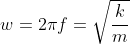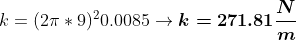---------------------------------------------------------------------

Energy: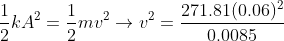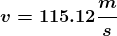-----------------------------------------------------------------------

Generel solution to problem: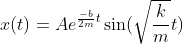for t=1.78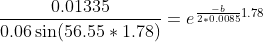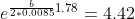Apply log to both sides: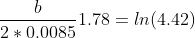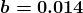#### Earn Coin

Coins can be redeemed for fabulous gifts.

Similar Homework Help Questions
• ### Block and Spring in SHM with damping Due this Friday, Apr 19 at 11:59 pm (EDT)...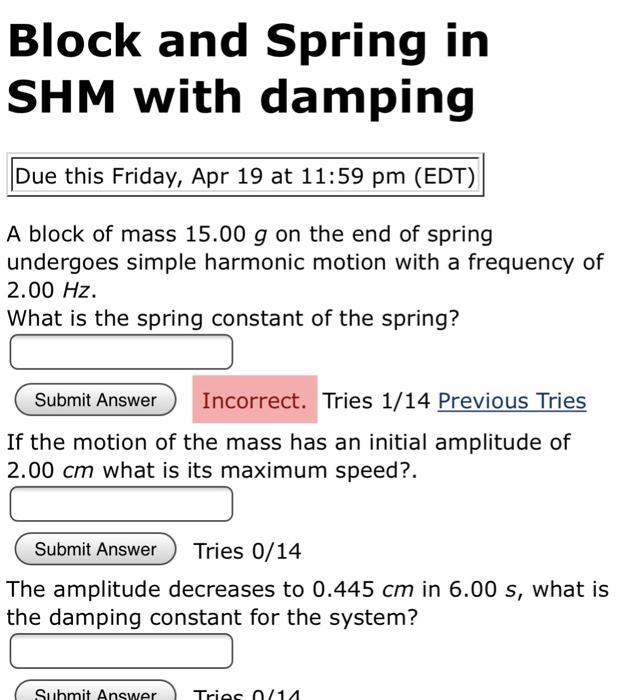Block and Spring in SHM with damping Due this Friday, Apr 19 at 11:59 pm (EDT) A block of mass 15.00 g on the end of spring undergoes simple harmonic motion with a frequency of 2.00 Hz. What is the spring constant of the spring? Submit Answer Incorrect. Tries 1/14 Previous Tries If the motion of the mass has an initial amplitude of 2.00 cm what is its maximum speed?. Submit AnswerTries 0/14 The amplitude decreases to 0.445 cm in...

• ### Block and Spring irn SHM with damping Due this Friday, Apr 20 at 11:59 pm (EDT)...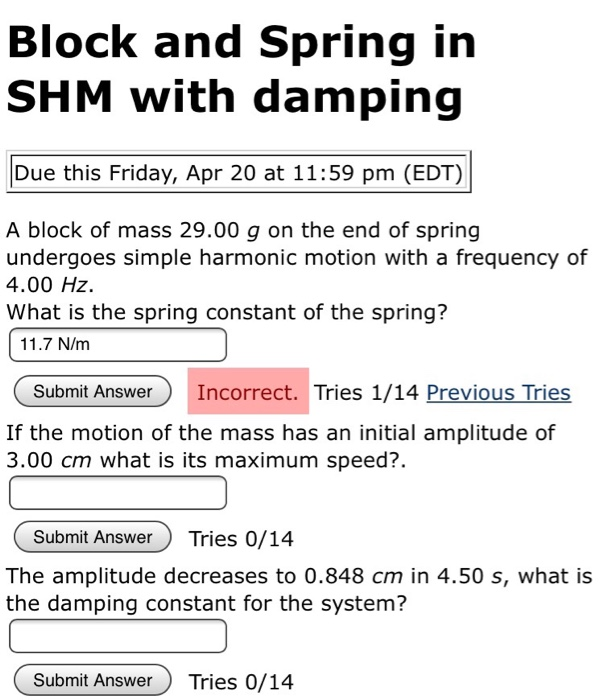Block and Spring irn SHM with damping Due this Friday, Apr 20 at 11:59 pm (EDT) A block of mass 29.00 g on the end of spring undergoes simple harmonic motion with a frequency of 4.00 HZ What is the spring constant of the spring? 11.7 N/m Submit AnswerIncorrect. Tries 1/14 Previous Tries If the motion of the mass has an initial amplitude of 3.00 cm what is its maximum speed?. Submit Answer Tries 0/14 The amplitude decreases to 0.848...

• ### Problem3 A (2+0.1y) kg block attached to a spring undergoes simple harmonic motion described by x...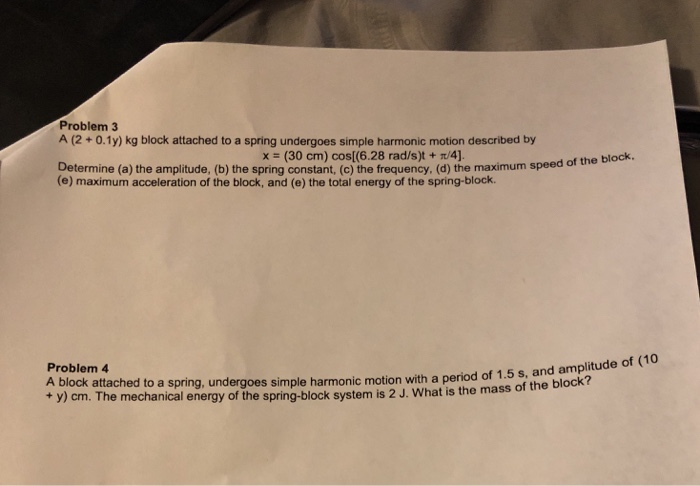Can you please answer both questions, Y=0 Problem3 A (2+0.1y) kg block attached to a spring undergoes simple harmonic motion described by x (30 cm) cos[(6.28 rad/s)t + /4) Determine (a) the amplitude, (b) the spring constant, (c) the frequency, (d) the maximum speed (e) maximum acceleration of the block, and (e) the total energy of the spring-block. of the block Problem 4 A block attached to a spring, undergoes simple harmonic motion with a period of 1.5 + y)...

• ### z waqod A 2- kg block attached to a spring undergoes simple harmonic motion described by...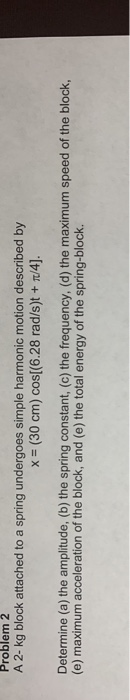z waqod A 2- kg block attached to a spring undergoes simple harmonic motion described by = (30 cm) cos[(6.28 rad/s)t + /4]. Determine (a) the amplitude, (b) the spring constant, (c) the frequency, (d) the maximum speed of the block, (e) maximum acceleration of the block, and (e) the total energy of the spring-block. Problem 3 A block attached to a spring, undergoes simple harmonic motion with a period of 1.5 s, and amplitude of 20 cm. The mechanical...

• ### 2. A block of unknown mass is attached to a spring with a spring constant of...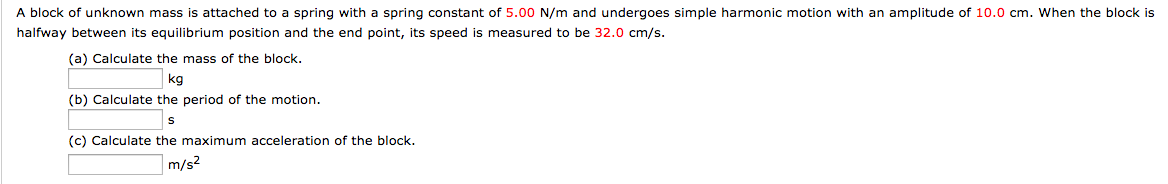2. A block of unknown mass is attached to a spring with a spring constant of 5.00 N/m and undergoes simple harmonic motion with an amplitude of 10.0 cm. When the block is halfway between its equilibrium position and the end point, its speed is measured to be 32.0 cm/s (a) Calculate the mass of the block (b) Calculate the period of the motion (c) Calculate the maximum acceleration of the block. kg m/s

• ### A wooden block (m = 0.640 kg) is connected to a spring and undergoes simple harmonic...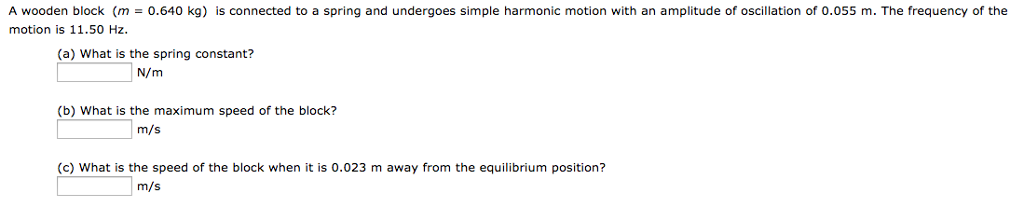A wooden block (m = 0.640 kg) is connected to a spring and undergoes simple harmonic motion with an amplitude of oscillation of 0.055 m. The frequency of the motion is 11.50 Hz. (a) What is the spring constant? N/m (b) What is the maximum speed of the block? m/s (c) What is the speed of the block when it is 0.023 m away from the equilibrium position? m/s

• ### A horizontal mass-spring system consists of a block (m=1.5 kg) on a frictionless to connected to...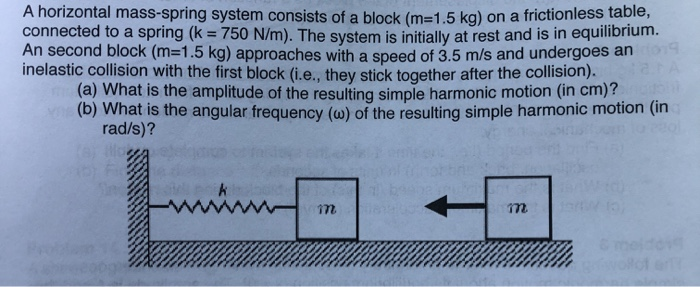A horizontal mass-spring system consists of a block (m=1.5 kg) on a frictionless to connected to a spring (k = 750 N/m). The system is initially at rest and is in equilibrium MI Second DIOCK (M=1.5 kg) approaches with a speed of 3.5 m/s and undergoes all inelastic collision with the first block (i.e.. they stick together after the collision). (a) What is the amplitude of the resulting simple harmonic motion (in cm)? (b) What is the angular frequency (w)...

• ### 15) In an electric shaver, the blade moves back and forth over a stance of 2.0 mm in simple harmo...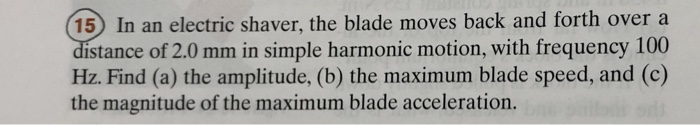15) In an electric shaver, the blade moves back and forth over a stance of 2.0 mm in simple harmonic motion, with frequency 100 Hz. Find (a) the amplitude, (b) the maximum blade speed, and (c) the magnitude of the maximum blade acceleration. 20 An oscillating block-spring system has a mechanical energy of 2.00 J, an amplitude of 10.0 cm, and a maximum speed of 0.800 m/s. Find (a) the spring constant, (b) the mass of the block, and (c)...

• ### 5. A mass of 225 g is suspended from a vertical spring. It is then pulled...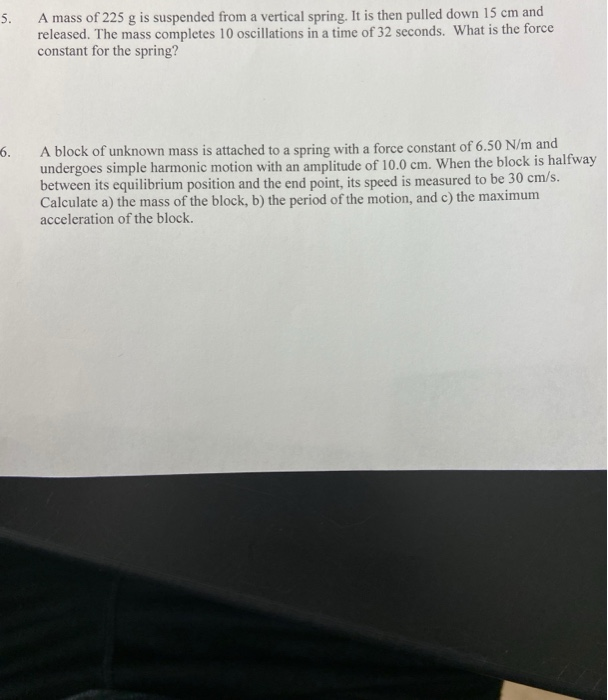5. A mass of 225 g is suspended from a vertical spring. It is then pulled down 15 cm and released. The mass completes 10 oscillations in a time of 32 seconds. What is the force constant for the spring? 6. A block of unknown mass is attached to a spring with a force constant of 6.50 N/m and undergoes simple harmonic motion with an amplitude of 10.0 cm. When the block is halfway between its equilibrium position and the...

• ### 2. Following problem 1, the same spring-mass is oscillating, but the friction is involved. The spring-mass starts oscillating at the top so that its displacement function is x Ae-yt cos(wt)...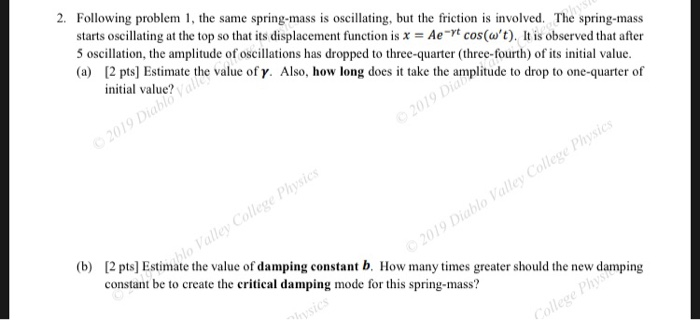2. Following problem 1, the same spring-mass is oscillating, but the friction is involved. The spring-mass starts oscillating at the top so that its displacement function is x Ae-yt cos(wt)t is observed that after 5 oscillation, the amplitude of oscillations has dropped to three-quarter (three-fourth) of its initial value. (a) 2 pts] Estimate the value ofy. Also, how long does it take the amplitude to drop to one-quarter of initial value? 0 Co [2 pts] Estimate the value of damping...